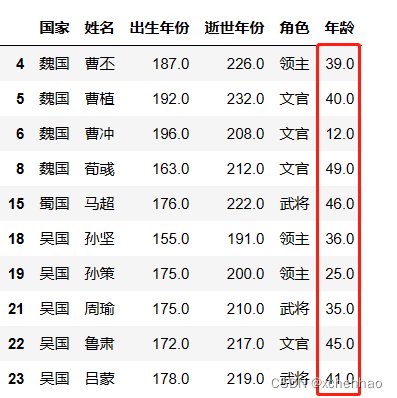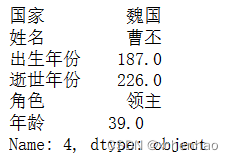﻿ pandas实战:分析三国志人物示例实现_python_脚本之家

# pandas实战:分析三国志人物示例实现

更新时间：2023年01月19日 10:52:58   作者：xchenhao

## 简介

### 背景

Pandas 是 Python 的一个工具库，用于数据分析。

Pandas 名称来源于 panel data（面板数据）和 Python data analysis（Python 数据分析）。

### 特点：

Series 和 DataFrame

（1）Series：一维数据（列+索引）

`pandas.Series(['东汉', '马腾', '?', 212], index=['国家', '姓名', '出生年份', '逝世年份'])`（2）DataFrame：二维数据（表格：多个列+行/列索引）```pandas.DataFrame([
['东汉', 300],
['魏国', 800],
['蜀国', 400],
['吴国', 600],
['西晋', 1000]
], columns=['国家', '国力'])```### 安装

`conda install pandas`

`pip install pandas`

`\$ head sanguo.csv`（1）导入模块

`import pandas as pd`

（2）读取 csv 数据

```# 当前目录下的 sanguo.csv 文件，na_values 指定哪些值为空
df = pd.read_csv('./sanguo.csv', na_values=['na', '-', 'N/A', '?'])```

1）查看数据

```# 查看前 5 条
# NaN 为空值``````# 查看后 5 条
df.tail(5)```2）查看数据概况

```df.dtypes
# 查看数据类型``````df.info()
# 有 25 行，5 列
# 各列的名称（kindom、name、birth、die、character）、非空数目、数据类型``````df.describe()
# 查看数值型列统计值：总数、平均值、标准差、最小值、25%/50%/75% 分位数、最大值```3）数据操作

```df.columns = ['国家', '姓名', '出生年份', '逝世年份', '角色']```# 计算年龄
df['年龄'] = df['逝世年份'] - df['出生年份']```# 筛选年轮小于 50 的数据
df[df['年龄'] < 50]``````# 筛选曹姓的数据
df[df['姓名'].str.startswith('曹')]``````df.groupby('国家')['姓名'].count()
# 类似于 SQL: SELECT 国家, COUNT(姓名) FROM x GROUP BY 国家```apply 函数

```df['状态'] = df['年龄'].apply(lambda x: '长寿' if isinstance(x, (int, float)) and x > 50 else '一般')`df.loc``df.loc[4:5]`df.loc[4, '姓名']df.iloc[4, 1]取第 5 行姓名列或第 5 行第 2 列df.loc[4, ['姓名', '年龄']] df.iloc[4, [1, 5]]取第 5 行姓名、年龄列或第 5 行第 2 列、第 6 列df.loc[4:5, ['姓名', '年龄']]df.iloc[[4, 5], [1, 5]]df.iloc[4:6, [1, 5]]取第 5~6 行姓名、年龄列或取第 5~6 行第 2 列、第 6 列df.iloc[4:9, 1:4]取 5~10 列第 2~5 列concat

```# 创建列
newpeople = pd.Series(['东汉', '马腾', '?', 212, '?'], index=['国家', '姓名', '出生年份', '逝世年份', '年龄'])

# 将 Series 转为 DataFrame，并对 DataFrame 转置（列转行）
newpeople = newpeople.to_frame().T

# 追加行（axis=0），重置索引（ignore_index=True）
df2 = pd.concat([df, newpeople], axis=0, ignore_index=True)
df2.tail()```merge

```# 创建表格
kindom_power = pd.DataFrame([
['东汉', 300],
['魏国', 800],
['蜀国', 400],
['吴国', 600],
['西晋', 1000]
], columns=['国家', '国力'])

# 按国家列进行两个表格（左 df，右 kindom_power）合并
df3 = pd.merge(left=df, right=kindom_power, on='国家')4）导出数据

```# 写入 sanguo_result.csv 中，不输出索引值
df.to_csv('sanguo_result.csv', index=False)```